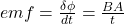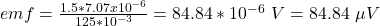## A magnetic field is uniform over a flat, horizontal circular region with a radius of 1.50 mm, and the field varies with time. Initially the

Question

A magnetic field is uniform over a flat, horizontal circular region with a radius of 1.50 mm, and the field varies with time. Initially the field is zero and then changes to 1.50 T, pointing upward when viewed from above, perpendicular to the circular plane, in a time of 125 ms. (a)What is the average induced emf around the border of the circular region? (Enter the magnitude in µV and the direction as seen from above.)

in progress 0
5 months 2021-08-13T17:30:41+00:00 1 Answers 64 views 0

The average induced emf around the border of the circular region is 84.84 μV

Explanation:

Given;

radius of the circular region, r = 1.50 mm

change in magnetic field strength, B =  1.50 T

time of change in magnetic field strength, t = 125 ms

the average induced emf around the border of the circular region:where;

A is the area of the circular region = πr²

A = π(1.5 x 10⁻³)² = 7.07 x 10⁻⁶ m²

substitute in the Value of A in the equation above;Therefore, the average induced emf around the border of the circular region is 84.84 μV